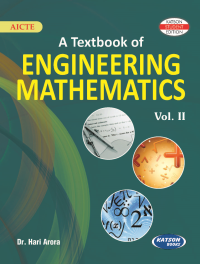•## A Textbook of Engineering Mathematics-II (AICTE)

In Stock
ISBN 978-93-5014-685-9 Dr. Hari Arora S.K. Kataria & Sons 1st 2019 2019 750 Print In Stock
Rs 695.00Rs 626.00

#### CONTENTS

• First Order Ordinary Differential Equations
• Ordinary Differential Equations of Higher Orders
• Multivariable Calculus (Integration)

• Vector Differential Calculus
• Vector Integral Calculus
• Matrices

• Sequences and Series
• Complex Variable Differentiation
• Complex Variable Integration
• Laplace Transforms
• Fourier Transforms
• Partial Differential Equations
• Index.

• Author : Dr. Hari Arora
• Publisher : S.K. Kataria & Sons
• Edition : 1st 2019
• Publishing Year : 2019
• Total Pages : 750